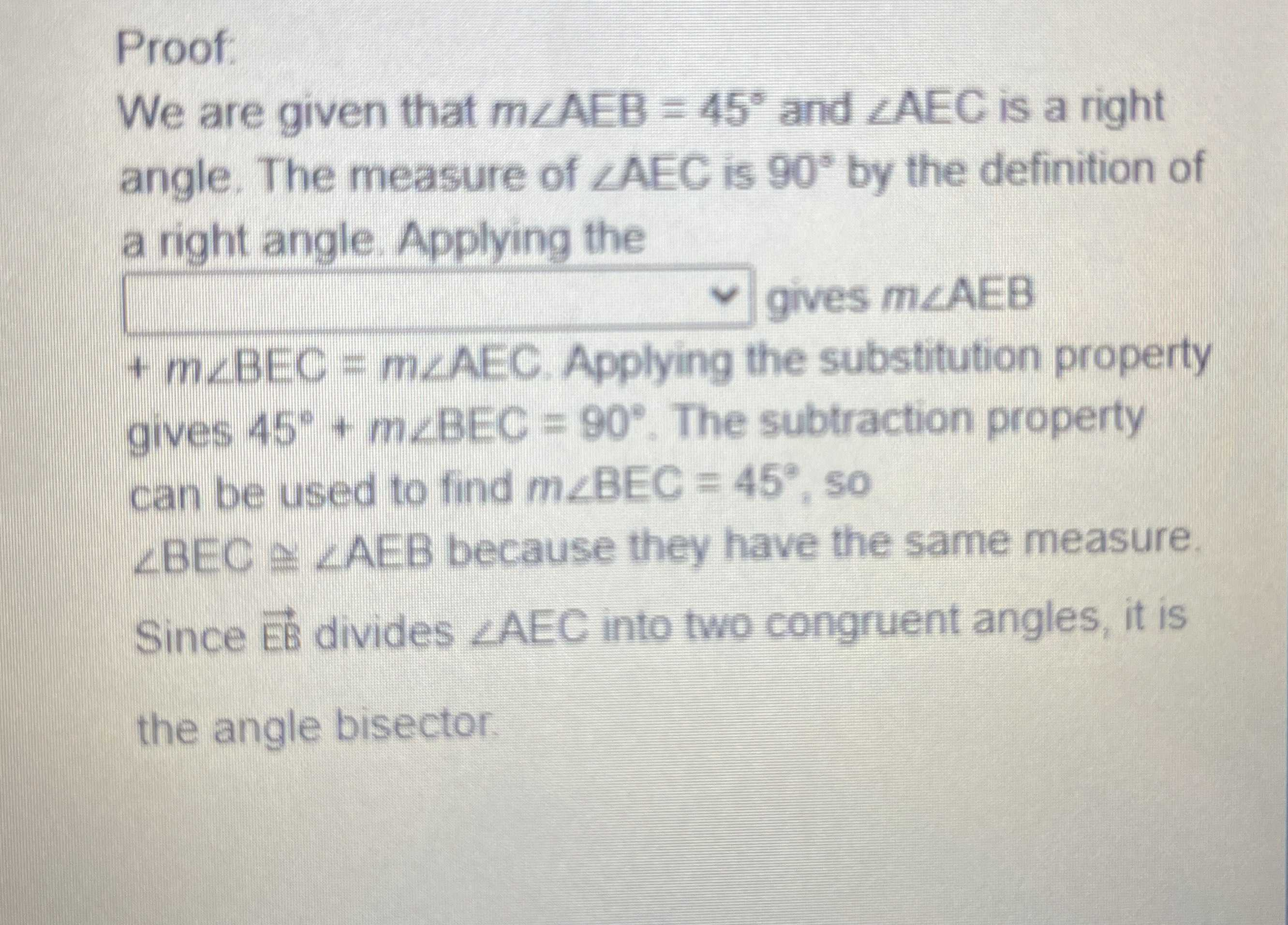### Still have math questions?

Trigonometry
QuestionProof: We are given that $$m \angle AEB = 45 ^ { \circ }$$ and $$\angle AEC$$ is a right angle. The measure of $$\angle AEC$$ is $$90 ^ { \circ }$$ by the definition of a right angle. Applying the

$$+ m \angle B E C = m \angle A E C$$ . Applying the substitution property gives $$45 ^ { \circ } + m \angle B E C = 90 ^ { \circ }$$ . The subtraction property can be used to find $$m \angle B E C = 45 ^ { \circ }$$ , so

$$\angle B E C \cong \angle A E B$$ because they have the same measure. Since $$\vec { E B }$$ divides $$\angle A E C$$ into two congruent angles, it is the angle bisector.